# Test: Fractions And Decimals - 3

## 20 Questions MCQ Test Mathematics (Maths) Class 7 | Test: Fractions And Decimals - 3

Description
This mock test of Test: Fractions And Decimals - 3 for Class 7 helps you for every Class 7 entrance exam. This contains 20 Multiple Choice Questions for Class 7 Test: Fractions And Decimals - 3 (mcq) to study with solutions a complete question bank. The solved questions answers in this Test: Fractions And Decimals - 3 quiz give you a good mix of easy questions and tough questions. Class 7 students definitely take this Test: Fractions And Decimals - 3 exercise for a better result in the exam. You can find other Test: Fractions And Decimals - 3 extra questions, long questions & short questions for Class 7 on EduRev as well by searching above.
QUESTION: 1

### Which of the following is true with respect to 9/16 and 13/5?

Solution:

L.C.M. is 16×5 =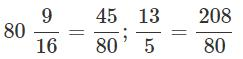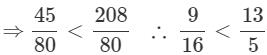QUESTION: 2

### Which of the following is the improper fraction form of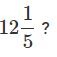Solution: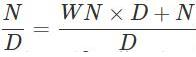where W is the whole number, N is the numerator and D is the denominator. So,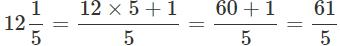QUESTION: 3

### What is the sum of 3/4,7/6  and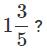Solution:

To find the sum of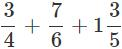Find the L.C.M. of the denominators and then add them. L.C.M. of 4, 5 and 6 is 60.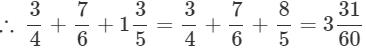QUESTION: 4

What is the difference 13/15 and 11/35 ?

Solution: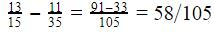QUESTION: 5

Which of the following is a proper fraction?

Solution:
QUESTION: 6

What is the product of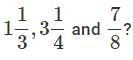Solution: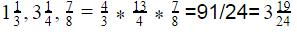QUESTION: 7

Guru reads 3/5 of a book. He finds that there are still 80 pages left to be read. What is the total number of pages in the book?

Solution:

No. pages left =(1−3/5)×  total pages
∴Total number of pages = 80× 5/22 = 200

QUESTION: 8

What is 1/7 of 49 litres?

Solution:

1/7× 49  litres and 7 litres.

QUESTION: 9

Indian cricket team won 4 more matches than it lost with New Zealand. If it won 3/5 of its matches, how many matches did India play?

Solution:

Let the number of matches lost hex. The number of matches won = x+4.
Total matches played  = x+x+4
We have,  x+4 = 3/5 (2x+4) ⇒ x = 8
∴  Total matches played = 20

QUESTION: 10

What is the product of a fractional number and its reciprocal?

Solution:

The product of a fraction and its reciprocal is always 1.

In the case of a whole number, think of it as having a denominator of 1: The reciprocal of 5 is 1/5.

multiplying 5 and 1/5 gives 1.

Every number has a reciprocal except for 0.

QUESTION: 11

What should be added to 21/27  to make it 26/27 ?

Solution:
QUESTION: 12

What are fractions with different denominators called?

Solution:
QUESTION: 13

What is the equivalent fraction of 8/11 having the numerator 40?

Solution:
QUESTION: 14

What do you call the fractions which have one as the numerator?

Solution:
QUESTION: 15

In which of the following fractions is the numerator greater than the denominator?

Solution:
QUESTION: 16

1/9 of a number is 5.What is the number?

Solution:

Let 'x' be the number. 1/9  of  x = 5 ⇒ x = 5×9 = 45

QUESTION: 17

Given that P/q = s/t, which of these is true?

Solution:

P/q = s/t ⇒ pt = sq (By cross multiplication.)

QUESTION: 18

What should be added to 3 to get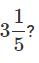Solution: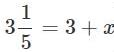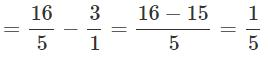QUESTION: 19

If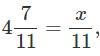what is the value of x?

Solution: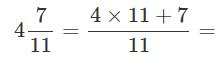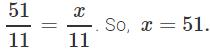QUESTION: 20

What is the lowest form of 12/30 ?

Solution:

12/30 = 2/5 is the required lowest form.